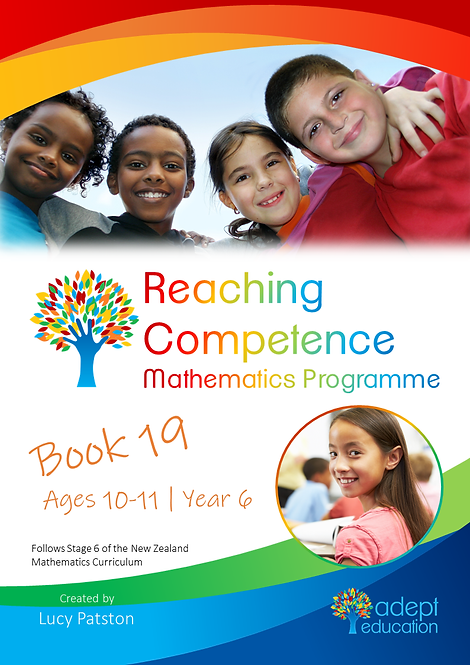`Books 18, 19 and 20 cover 27 learning intentions for children in Year 6, aged about 10 to 11 years old. At this level, students are learning to choose the best way to solve an addition or subtraction problem from a range of strategies. They see numbers as ‘whole’ units but also understand that ‘nested’ within these units is a range of possibilities for partitioning (breaking up) and recombining. This is called part-whole thinking.For example, 29 + 28 = ?Using compensation: 30 + 27 or 30 + 28 – 1Using place value partitioning: 29 + 20 + 8or 20 + 20 + 9 + 8.They can also derive multiplication from known facts, e.g., 3 x 8 = 24, so 6 x 8 = 24 + 24 = 48. By now they should know their times tables, decimal numbers to three places and be able to read any fraction. Get this 94-page activity book in PDF format immediately upon purchase. View the sample of Book 19 here`

# Book 19 e-Copy 2021 Edition

\$8.95Price
• Book 19 covers square numbers, division and multiplication, and fractions and ratios.

​For students who have a sound understanding of multiplication/division and fractional concepts.

Book 19 covers some of Stage 6. Together, Books 18, 19 and 20 cover all of Stage 6.

You will also get: